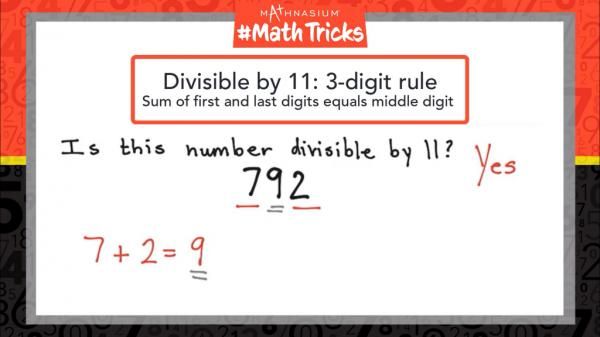* indicates a required field• phone number format invalid• email format invalidProblems detected, please review the form.
Privacy - Terms

News from Mathnasium of Tyrone

Mathnasium #MathTricks: Divisibility (3-digit Rule for 11s)

Nov 3, 2021One way to test if a three-digit number is divisible by 11 is to follow the 3-digit rule: the sum of the first and last digits equals the middle digit.

To determine if the number 792 is divisible by 11, we find the sum of seven and two. Seven plus two gives us a sum of nine, which is equal to the middle digit, nine. So,

792 is divisible by 11.